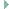bit.h File Reference

Bit access macros for Cortex-M based CPUs. More...

## Detailed Description

Bit access macros for Cortex-M based CPUs.

Definition in file bit.h.

`#include <stdint.h>`
`#include "cpu.h"`Include dependency graph for bit.h:

Go to the source code of this file.

 #define CPU_HAS_BITBAND   1 || 0 (1 for Cortex-M3 and up, 0 for Cortex-M0) Flag for telling if the CPU has hardware bit band support.

## Bit manipulation functions

static volatile void * bitband_addr (volatile void *ptr, uintptr_t bit)
Convert bit band region address and bit number to bit band alias address. More...

static void bit_set32 (volatile uint32_t *ptr, uint8_t bit)
Set a single bit in the 32 bit word pointed to by `ptr`. More...

static void bit_set16 (volatile uint16_t *ptr, uint8_t bit)
Set a single bit in the 16 bit word pointed to by `ptr`. More...

static void bit_set8 (volatile uint8_t *ptr, uint8_t bit)
Set a single bit in the 8 bit byte pointed to by `ptr`. More...

static void bit_clear32 (volatile uint32_t *ptr, uint8_t bit)
Clear a single bit in the 32 bit word pointed to by `ptr`. More...

static void bit_clear16 (volatile uint16_t *ptr, uint8_t bit)
Clear a single bit in the 16 bit word pointed to by `ptr`. More...

static void bit_clear8 (volatile uint8_t *ptr, uint8_t bit)
Clear a single bit in the 8 bit byte pointed to by `ptr`. More...

## ◆ bit_clear16()

 static void bit_clear16 ( volatile uint16_t * ptr, uint8_t bit )
inlinestatic

Clear a single bit in the 16 bit word pointed to by `ptr`.

The effect is the same as for the following snippet:

*ptr &= ~(1 << bit);

There is a read-modify-write cycle occurring within the core, but this cycle is atomic and can not be disrupted by IRQs

Parameters
 [in] ptr pointer to target word [in] bit bit number within the word

Definition at line 163 of file bit.h.

## ◆ bit_clear32()

 static void bit_clear32 ( volatile uint32_t * ptr, uint8_t bit )
inlinestatic

Clear a single bit in the 32 bit word pointed to by `ptr`.

The effect is the same as for the following snippet:

*ptr &= ~(1 << bit);

There is a read-modify-write cycle occurring within the core, but this cycle is atomic and can not be disrupted by IRQs

Parameters
 [in] ptr pointer to target word [in] bit bit number within the word

Definition at line 143 of file bit.h.

## ◆ bit_clear8()

 static void bit_clear8 ( volatile uint8_t * ptr, uint8_t bit )
inlinestatic

Clear a single bit in the 8 bit byte pointed to by `ptr`.

The effect is the same as for the following snippet:

*ptr &= ~(1 << bit);

There is a read-modify-write cycle occurring within the core, but this cycle is atomic and can not be disrupted by IRQs

Parameters
 [in] ptr pointer to target byte [in] bit bit number within the byte

Definition at line 183 of file bit.h.

## ◆ bit_set16()

 static void bit_set16 ( volatile uint16_t * ptr, uint8_t bit )
inlinestatic

Set a single bit in the 16 bit word pointed to by `ptr`.

The effect is the same as for the following snippet:

*ptr |= (1 << bit);

There is a read-modify-write cycle occurring within the core, but this cycle is atomic and can not be disrupted by IRQs

Parameters
 [in] ptr pointer to target word [in] bit bit number within the word

Definition at line 103 of file bit.h.

## ◆ bit_set32()

 static void bit_set32 ( volatile uint32_t * ptr, uint8_t bit )
inlinestatic

Set a single bit in the 32 bit word pointed to by `ptr`.

The effect is the same as for the following snippet:

*ptr |= (1 << bit);

There is a read-modify-write cycle occurring within the core, but this cycle is atomic and can not be disrupted by IRQs

Parameters
 [in] ptr pointer to target word [in] bit bit number within the word

Definition at line 83 of file bit.h.

## ◆ bit_set8()

 static void bit_set8 ( volatile uint8_t * ptr, uint8_t bit )
inlinestatic

Set a single bit in the 8 bit byte pointed to by `ptr`.

The effect is the same as for the following snippet:

*ptr |= (1 << bit);

There is a read-modify-write cycle occurring within the core, but this cycle is atomic and can not be disrupted by IRQs

Parameters
 [in] ptr pointer to target byte [in] bit bit number within the byte

Definition at line 123 of file bit.h.